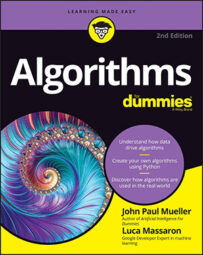##### Algorithms For DummiesAlgorithms are fun! Algorithms are beautiful! Algorithms are even better than your favorite pastime! Well, perhaps not the last one. In fact, algorithms surround you in many ways you might not have thought about, and you use them every day to perform important tasks.

However, you need to be able to use algorithms in a way that doesn’t involve becoming a mathematician. Programming languages make it possible to describe the steps used to create an algorithm, and some languages are better than others at performing this task so that people can understand it without becoming a computer or data scientists. Python makes using algorithms easier because it comes with a lot of built-in and extended support (through the use of packages, datasets, and other resources).

With that in mind, this Cheat Sheet helps you access the most commonly needed tips for making your use of algorithms fast and easy.

## Locating the algorithm you need

The following table describes algorithms and algorithm types that you might find useful for various types of data analysis.

## Differentiating algorithms from other math structures

If you’re like most people, you often find yourself scratching your head when it comes to math structures because no one seems to know how to use the terms correctly. It’s as though people are purposely trying to make things hard! After all, what is an equation and why is it different from an algorithm?

Well, fear no more: The following table provides the definitive guide to math structures that you might encounter but have been afraid to ask about.

 Structure Description Equation Numbers and symbols that, when taken as a whole, equate to a specific value. An equation always contains an equals sign so that you know that the numbers and symbols represent the specific value on the other side of the equals sign. Equations generally contain variable information presented as a symbol, but they aren’t required to use variables. Formula A combination of numbers and symbols used to express information or ideas. A formula normally presents mathematical or logical concepts, such as to define the Greatest Common Divisor (GCD) of two integers (this Kahn Academy video tells how this works). Generally, a formula shows the relationship between two or more variables. Most people see a formula as a special kind of equation. Algorithm A sequence of steps used to solve a problem. The sequence presents a unique method of addressing an issue by providing a particular solution. An algorithm need not represent mathematical or logical concepts, even though the presentations in this book often do fall into that category because people most commonly use algorithms in this manner. Some special formulas are also algorithms, such as the quadratic formula. For a process to represent an algorithm, it must be the following: Finite: The algorithm must eventually solve the problem. Well-defined: The series of steps must be precise and present steps that are understandable, especially by computers, which must be able to create a usable algorithm. Effective: An algorithm must solve all cases of the problem for which someone defined it. An algorithm should always solve the problem it has to solve. Even though you should anticipate some failures, the incidence of failure is rare and occurs only in situations that are acceptable for the intended algorithm use.

## Amazing ways to use algorithms

You have likely used an algorithm today without knowing it, as have most other people. For example, making toast is an example of an algorithm, as explained in this blog post. Making toast isn’t an amazing algorithm, but the ones in the following table, which use a computer to perform tasks, are.

## Dealing with algorithm complexity

Time is money, which may not always mean what you think it means (see this blog post by Opher Ganel). However, time complexity in algorithms does generally break down into lost time, use of additional resources, and, yes, money.

One way to compare two algorithms is through time complexity. You need to know how complex an algorithm is, because the more complex is the algorithm, the longer it takes to run. However, time complexity isn’t the only comparison measure. If one algorithm takes twice as long to run but produces a dependable result three times as often as another algorithm that runs in half the time, you may need to use the slower algorithm.

The following table helps you understand the various levels of time complexity presented in order of running time (from fastest to slowest).